All Exams Free Study Material and PDFs Join Our Telegram Channel Click Here Join Now

# Mixtures & Alligations Tricks & Tips

Mixture & Alligation Short-Cut Tricks & Tips : Fillers Short-Cut Tricks & Tips Question Pdf for Banking, SSC, RRB, FCI, Railway, UPSC, State PCS, Insurance & other Competitive exams. Mixture & Alligation Short-Cut Tricks & Tips shortcut Tricks Pdf, Mixture & Alligation Short-Cut Tricks & Tips MCQ, Mixture & Alligation Short-Cut Tricks & Tips Objective Question & Answer Pdf. “Fillers Short-Cut Tricks & Tips Questions PDF” In this post we are providing you the Mixture & Alligation Short-Cut Tricks & Tips pdf with detailed solution & Short Tricks. So that you can easily get the logic of question. This Mixture & Alligation Short-Cut Tricks & Tips Pdf we are Providing is free to download. ” Most Important Fillers Short-Cut Tricks & Tips Question PDF with Answers

Mixture & Alligation Short-Cut Tricks & Tips Plays a vital role in Exam. In every exam you will get at least 5-10 questions from this topic. So candidates must focus on this topic and download this Mixture & Alligation Short-Cut Tricks & Tips pdf to get important questions with best solution regarding Mixture & Alligation Short-Cut Tricks & Tips. We have put all Previous Year Questions of Mixture & Alligation Short-Cut Tricks & Tips that are Asked in various Govt & Private Exam.

## INTRODUCTION TO MIXTURE AND ALLIGATION:

Hi Aspirants today we are going to discuss on a topic Mixture and Alligation. This  topic carries 1 mark in prelims and 2 mark in mains exam.This topic can be implemented in Topics such as Time and Distance ,Ratio and Proportions.

### MIXTURE:

When two or more components are mixed together then it is known as Mixture

Mixture is of two types they are:

1.Simple Mixture:When two or more different ingredients are mixed together,a simple mixture is formed

2.Compound Mixture:When two or more simple mixtures are mixed together, a compound mixture is formed.

### ALLIGATION:

It enables us to find the ratio in which two or more ingredients at the given price must be mixed to produce a mixture  of desired price.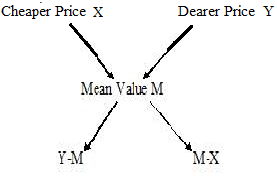Where M=Mean Value which is between X and Y, X=Cheaper Price, Y=Price higher than the Mean Value

## #.1.TYPE 1:

### MIXTURE CONTAINING TWO OR MORE INGREDIENTS:

#### 1.A Shopkeeper Sells Rice Of 1kg For Rs.75 W Two Quality Of Rice Whose Worth For 1kg Is Rs.70 And Rs.80.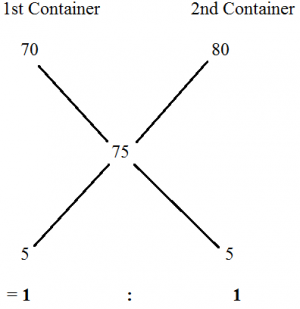Therefore for 1kg of rice the two quality of rice should be mixed in the ratio  1:1

## TYPE  2:

### BASED ON PROFIT OR LOSS:

#### 1.A Dishonest  Milkman Professes To Sell His  Milk At Cost Price But He Mixes It With Water And Thereby Gains 25%.The Percentage Of Water In The Mixture?

Let the Cost Price of  Pure Milk be Rs.1,

Selling Price of (Milk+ Water) is also Rs.1. which is 25% more than the Costprice

Therefore CostPrice = (100/125)*1=(4/5)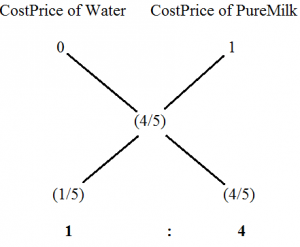The Percentage   of water mixed in the mixture =*100=20%

#### 2.The Ratio ,In Which Tea Costing Rs.192 Per Kg Is To Be Mixed  With Tea  Costing Rs.150 Per Kg So That The Mixed Tea  When  Sold For Rs.194.40 Per Kg,Gives A Profit Of 20%.

 Quality Cost Price Selling Price Selling Price after Profit/Loss 1st Quality 150 194.40  after 20% profit 2nd Quality 192

Finding   Selling Price after Giving Profit over Cost Price:

Let the Cost Price be X

Selling Price=X*(120/100)  = 194.40

X=(19440/120)  X=162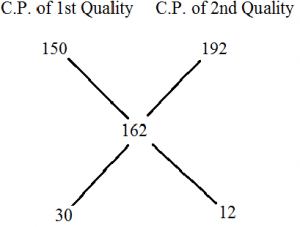30:12           5:2

The 1st and 2nd quality of rice should be mixed in the ratio:5:2

Remember:

If there is a loss in the transaction indicate it by the ‘-‘ negative sign before the number.

## TYPE 1:

### BASED  ON THREE INGREDIENTS OR THREE RATIO’S:

1.The Grocer sells apples of 3 varieties whose price per kg’s are Rs.40,Rs.60  and Rs. 90 .If he sells 1kg of apple by missing all these 3 varieties for Rs.80 what is the ratio by which these varieties are to be mixed?

Explanation: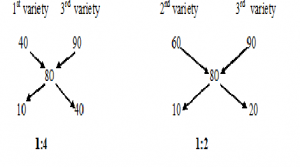1st variety 3rd variety 2nd  variety 1 4 2 1

Ratio’s by which these 3 Qualities are missed are=1:2:4

2.Tea worth Rs.126   per kg and Rs.135 per kg are mixed with a third variety in the ratio 1:1:2.If the mixture is worth Rs.153 per kg, the price of the third variety per kg will be?

Explanation:

Given that the tea worth Rs.126 and Rs.135 per kg  is mixed in the ratio 1:1.

Therefore price for 1kg=  = Rs.130.50

Now we have two price 130.50 and the price of the third variety is X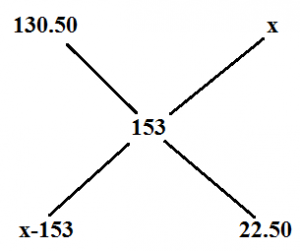The price of the third variety=Rs.175.50

3.There are three vessels of equal capacity holds milk and water in the ratio of 1:2,2:3 and 1:4if the content of all the three vessel s are mixed in asingle vessel then.Find the ratio of milk and water in the new vessel?

Explanation:

Here there are three ratio’s

1:2=3Part

2:3=5Part

1:4=5Part

Lcm =15Litres

 Milk Water 5Litres 10Litre 6Litre 9Litre 3Litre 12Litre 14Litres 31Litre

The new Ratio is 14:31

## TYPE 2:

### BASED ON REMOVING AND ADDING SOME QUANTITY :

1)How much water must be added to 60 litres of milk at 1.5 litre for Rs.20,So as to have a mixture worth Rs.10 2/3 per litre?

Explanation:

C.P for 1.5 litre of milk =Rs.20

C.P  for 1 litre of milk=Rs.40/3

C.P for 1 litre of water=Rs.0

Given Mean value=Rs.32/3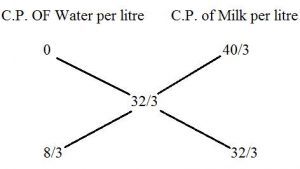8/3  :   32/3= 1:4

Therefore water in the mixture in 60 litres is (1/5)*60=12 litres

2)In a 729 litres mixture of milk and water ,the ratio  of milk to water is 7:2.To get a new mixture containing milk and water in the ratio 7:3,the amount of water to be added is?

Explanation:
 729 7x 2x

9x=729           x=81

Old Mixture     New Mixture

7:2                       7:3

Already the mixture contains water in the proportion 2x  and the new mixture contains water in the proportion 3x .So x quantity of water is added  to the mixture .Therefore 81 litres of water is added to the new mixture.

3)8 litres are drawn from a cask filled with wine and is then filled with water .This operation is performed three more times.The ratio of the quantity of wine now left in the cask to that of the total solution is 16:81.How much wine does the cask originally ?

Explanation:

When the final amount of solute that is not replaced calculated as:

Initial Amount * (Volume After Removal/Volume After Replacing)^n

Final  ratio of solute not replaced to total is

Initial Ratio * (Volume After Removal/Volume After Replacing)^n

Here let us assume the initial value is 1 then Wine .Let the Quantity of wine in the cask originally be x

Therefore

1* [(x-8)^4/x]

=[16/81]

x=24litres

Wine originally in the cask is 24 Litres

4)A vessel contains mixture of liquids A and B in the ratio 3:2.When 20 litres of the mixture  is taken out and replaced by 20litres of liquid B,the ratio changes to 1:4.How many litres of liquid A was there initially present in the vessel?

Explanation:

Original ratio=3:2

3/5 * (5X-20)/5x=1/4

X=6  then the liquid A in the vessel =3X=3*6=18Litres

### IMPORTANCE OF MIXTURE AND ALLIGATION:

Mixture and Alligation is used in other Miscellaneous Topic such as

Ratio and Proportion

Time and Distance

You can reduce the time taken to solve the problem if you use Mixture and Alligation Method

### BASED ON ALLOYS:

1.A milk vendor has 2 cans of milk. The  first contains 25% water and he rest milk.The second container 50% water.How much milk should he mix from each of the containers so as to get 12 litres of milk such that the ratio of water to milk is 3:5?

Explanation:

Given that

Milk in 1st Can = 3/4

Milk in 2nd Can =1/2

We want milk in the ratio=5/8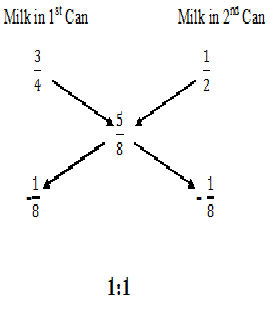(1/2)*12=6litres  So, quantity of mixture taken from each can=6litre

2.The milk and water in two vessels A and B are in the ratio 4:3 and 2:3  respectively .In what  ratio  the liquids in both vessels be mixed to obtain a new mixture in vessel c consisting half milk and  half water?

Explanation:

Mean Value=(1/2)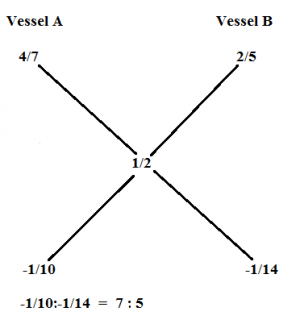Therefore Milk and Water should be mixed in the Ratio of 7:5

## #.2 TYPE 2:

### FINDING NUMBER  OF PERSONS :

1.In  a zoo there  are Rabbits and Pigeons.If heads are counted ,there are 200 and if legs are counted there are 580.How many pigeons are there?

Explanation:

Total Legs Count=580

Average Leg Count =580/200

29/10

Average leg Count for Rabbit =4,

Average leg Count for Pigeons=2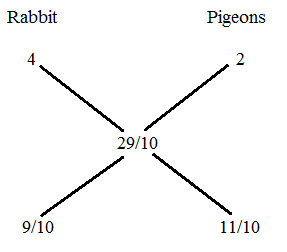9:11

Thus the number of Pigeons is  * 200=110 pigeons

2.The total number of people in the village is 5000.If the male and female increases by 10% and 15% respectively,the population of the village becomes 5600.What is the number of male in the village?

Explanation:

Total number of people in the village=5000

10% of the village =500   15% of  the village=750

Increased number of people=5600-5000=600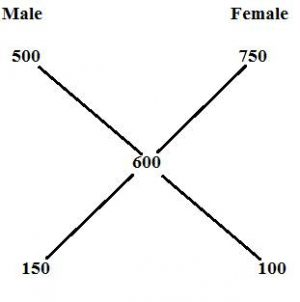=150:100

=3:2

Male in village=3000   ⇒     (5000*3/5=3000)

## #.3. TYPE 3:

### PARTIAL WORK:

1.A shopkeeper sells 1000kg of sugar ,part of which he sells at 8% profit and the rest at 18% profit .He gains 14% on the whole. The quantity sold at Rs.18% is?

Explanation: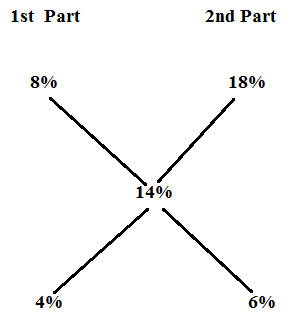4:6

=2:3

Therefore  the 1000kg  sugar is sold in the ratio  400:600

Sugar sold at the profit of 18%=600kg

TOPICS TO BE DISCUSSED

1)BASED ON FINDING INDIVIDUAL CAPACITY

2)BASED ON DIFFERENT CAPACITY

3)BASED ON RATIO’S

#### 1)A Jar Contains A Mixture Of Milk & Water In The Ratio 4:1.When 10 Litres Of The Mixture Is Taken Out & 10 Litres Of Water Is Poured Into The Jar,The Ratio Becomes 2:3.How Many Litres Of Milk Was In The Jar?

EXPLANATION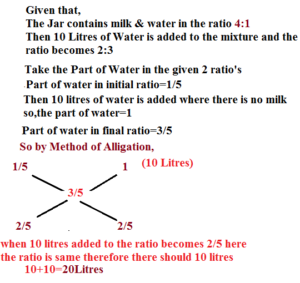### PRACTICE THIS SUM:

1)A Vessel Contains mixture of liquids A and B in the ratio 3:2.When 20 litres of the mixture is taen out and replaced by 20 litres of liquid B,the ratio changes to 1:4.How many litres of liquid A was there initially present in the vessel?

18 Litres

2)A can contains a mixture of two liquids in proportion 7:5.When 9 litres of mixture are drawn off and the can is filled with B,the proportion of A and B becomes 7:9.How many litres of liquid A was contained by the can initially?

21 Litres

## #.2.TYPE 2:

### BASED ON DIFFERENT CAPACITY:

1)Three glasses of Sizes 5 litres,10 litres & 15 litres contain mixture of Milk & Water in the ratio 3:2,4:6&4:11 respectively.The Contents of all the three glasses are poured into single vessel.Find the ratio of Milk to Water in the resultant mixture.

EXPLANATION

To find the Ratio of Milk to Water in the resultant Mixture,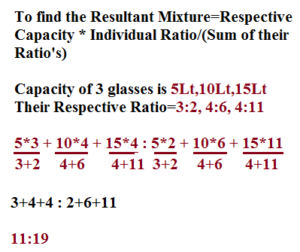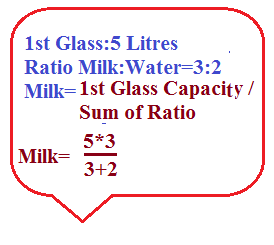### PRACTICE THIS SUM:

1)Three bottle of Capacity 6 Litres,12 Litres and 4 litres contain mixture of Sugar water and honey in the ratio 1:5 , 7:5 & 1:3 respectively.The contents of all the three bottles are poured into single bottle.Find the ratio of Sugar water to honey in the resultant mixture?

9:13

2)Four glasses of sizes 2Litres,8 Litres,12 Litres & 16 Litres contain mixture of milk & Water in the ratio 1:1 ,3:5,7:5,9:7 respectively.The Contents of all the four glasses are poured into single glass.Find the ratio of Milk & Water in the resultant Mixture?

The Resultant ratio=20:18⇒10:9

## #.3. TYPE 3:

### BASED ON RATIO’S:

1)In an Alloy,Zinc and Copper are in the ratio 3:4,In the Second alloy the same elements are mixed in the ratio 7:2.In what ratio should these two alloys be mixed to form a new alloy in which the two elements are in the ratio 5:6?

EXPLANATION

The ratio is written as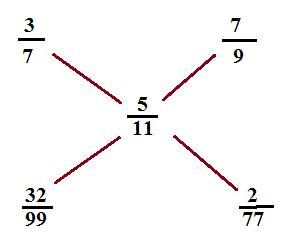The ratio is 32/99:2/77

### PRACTICE THIS SUM:

#### 1)In An Alloy The Zinc & Copper Are In The Ratio 1:3.In The Second Alloy The Same Elements Are In The Ratio 4:3.In What Ratio Should These Two Alloys Be Mixed To Form A New Alloy In Which The Two Elements Are In The Ratio 4:5?

The Resultant ratio=32:49

2)A jar full of lime juice contains juice and sugar water in the ratio 2:3.In the 2nd Jar the same elements are in the ratio 7:2.In what ratio should these two elements be mixed to for a new alloy in which the two elements are in the ratio 6:5?

The Resultant ratio=1165:232

“One Topic, to rule them all”

Yes…Mixture and Alligation has its own charm. A good hold of it can help you in solving a wide range of questions.

Alligation is a method of solving arithmetic problems related to mixtures of ingredients. Please note that alligation method is applied for percentage value, ratio, rate, prices, speed, etc. and not for absolute value. That is whenever per cent, per km, per hour, per kg, are being compared, we can use Alligation.

Common trick for Ratio-Proportion and Mixture Alligation : Almost 50% of the questions are solvable just by going through the options. Just go through the questions I have solved in this article and you will know the approach.

Rule of Alligation

Ingredient A : Ingredient B = M – Y : X – M

Here Mean Price is something which applies on the whole thing. If two varieties  of tea costing Rs. X and Rs. Y respectively are mixed and sold at Rs. Z, then Z is the mean price because it is price of the mixture.

Rule of Alligation

if the gradients are mixed in a ratio, thenWe represent it as under:Then, (cheaper quantity) : (dearer quantity) = (d – m ) : (m – c)

Q.1

Note that Rs. 180/kg and Rs. 280/kg are cost prices, while Rs. 320 is the selling price. To apply the alligation formula, all the three prices should be similar. So we will convert SP into CP
Given SP = Rs. 320/kg, Profit = 20%
Hence CP = 320/1.2 = Rs. 800/3
So the Mean price is Rs. 800/3 per kg
Now you can apply the formula-
Type 1 : Type 2 = 280 – 800/3 : 800/3 – 180 = 2 : 13

Both the containers have equal capacity. Let us assume that both containers are of 28 litres. Why 28? Because 28 is the LCM of (3 + 1) and (5 + 2) or 4 and 7. So taking the capacity as 28 litres will make your calculations easier.

In Container 1, we have (3/4)*28 = 21 litres of milk and (1/4)*28 = 7 litres of water.
In Container 1, we have (5/7)*28 = 20 litres of milk and (2/7)*28 = 8 litres of water.
Total milk in both the containers = 21 + 20 = 41
Total water in both the containers = 7 + 8 = 15
Milk : Water = 41 : 15
Shortcut
Container 1 has 3 times more milk than water
Container 2 has 2.5 times more milk than water
When the contents of the two containers are mixed, the milk will still be more than water. How much more ? Somewhere between 2.5 and 3 times
(D) is the only option where the quantity of milk is around 2.7 times (i.e. between 2.5 and 3) that of the water.

Milk in vessel A = 4/7
Milk in vessel B = 2/5
Milk in vessel C = 1/2 (because in vessel C, milk and water are present in 1:1 ratio)
You have to mix 4/7 and 2/5, to produce 1/2. Hence 1/2 is the Mean Price.
A : B = (1/2 – 2/5)/(4/7 – 1/2) = 14 : 10 = 7 : 5

Shortcut
Final ratio of the three varieties is 5 : 7 : 9
The question asks us the quantity of third variety of tea in the final mixture. From the above ratio, it is clear that the quantity of the third variety is a multiple of 9. So 45 is the only option possible.
Method
Let the three quantities be 4x, 5x and 8x
New quantities are 4x + 5, 5x + 10 and 8x + p
Now 4x + 5 : 5x + 10 : 8x + p = 5 : 7 : 9
(4x + 5)/(5x + 10) = 5/7 and (4x + 5)/(8x + p) = 5/9
Solving 1st equation, we get x = 5
Solving 2nd equation, we get p = 5
In the final mixture the quantity of the third variety is 8x + p = 8*5 + 5 = 45

In this question we will use the below formula

So from the above formula
(Quantity of acid left)/(Quantity of acid in the original mixture) = (1 – 4/20)^2 = 16:25

Let the original quantities of A and B be 4x and x
In 10 litres, quantity of A = 4/5 * 10 = 8 litres
In 10 litres, quantity of B = (10 – 8) = 2 litres
New quantities of A and B are 2x and 3x
(Original Quantity of A) – (New quantity of A) = 8 litres[Because after taking out 10 litres of the mixture, the quantity of liquid A reduced by 8 litres]
So, 4x – 2x = 8
or x = 4
Hence quantity of liquid A in original mixture = 4*4 = 16 litres
Note : In the above question, there were two different ratios 4:1 and 2:3, then too I took the same constant of proportionality for them, i.e. ‘x’ because the following two conditions were met:
1. The volume of mixture did not change (Like in this question 10 litres were replaced, not removed)
2. The two ratios had same no. of parts (4:1 and 2:3 both have 5 parts)
You can take different constant to solve the question, but that will make the calculations little lengthy.

Since the ratio of alcohol and water is 1:4, hence quantities of alcohol and water in the mixture are 3 litres and 12 litres respectively.
Total volume will become 18 litres after adding 3 litres water
% of alcohol = 3/18 * 100 = 50/3%

Q. 8)

Shortcut
Originally there are 1512 story books
Final ratio of Story books : Others = 15:4
That means the story books are a multiple of 15.
Just look at the options and see which number when added to 1512, will give a multiple of 15

Method
Let the no of story and other books be 7x and 2x respectively
Given 7x = 1512
x = 216
Now let the final quantity of story and other books be 15y and 4y respectively.
Since only story books are added to the collection, hence the quantity of other books has remained unchanged.
So 2x = 4y or y = x/2 = 108
We have to find 15y – 7x = 15y – 14y = y [Since x = 2y]

Let us solve some CGL questions that may not seem to belong to Mixture-Alligation category, but are easier to solve via Alligation formula…

Let the gain% of the remaining land be x%. Then
Given, 2/5 land is sold on loss and hence 3/5 land is sold on profit
So, Profit : Loss = 3:2
Hence 16 : (x – 10) = 3 : 2
16/(x – 10) = 3/2
or x = 62/3%

His average speed for the entire journey = 61/9 km/hour
Time taken on foot : Time taken on bicycle = (9 – 61/9)/(61/9 – 4) = 4:5
Time taken on foot = 4/9 * 9 = 4 hours
Hence distance travelled on foot = (Speed on foot * Time taken on foot) = 4*4 = 16 km

Alternate method
This method is equally easy.
Let the distance travelled on foot be X km. Then distance travelled on bicycle = (61 – X)
Now he travelled for 9 hours. Hence
X/4 + (61 – X)/9 = 9
Solve for X
You will get X = 16 km

Number of legs per head = 420/180 = 7/3
Now cows have 4 legs while hens have 2 legs
Cows : Hens = 1/3 : 5/3 = 1 : 5
Now total number of cows and hens = total number of heads = 180
Number of cows = 1/6 * 180 = 30

A : B = 3 : 4 and B: C = 4 : 5
A : B : C = 3 : 4 : 5
Average score of classes A, B and C =

The man spends 75% of his income, that means he saves 25%
Hence Expenditure : Saving = 75 : 25 = 3 : 1
Let the % increase in the savings be x.
I have written 3 : 1 as 30 : 10 so that (% increase in income – % increase in expenditure) could agree with 3 : 1 ratio. As we know 20 – 10 = 10, not 1.
So now from the figure we can see that  x – 20 = 30
Hence x = 50%

If whole money is invested at 6%, then interest = 6% of 1500 = Rs. 90
If whole money is invested at 5%, then interest = 5% of 1500 = Rs. 75
Hence, Money invested at 6% :  Money invested at 5% = 10 : 5 = 2 : 1
Money invested at 5% = 1/3 * 1500 = Rs. 500

# Practice Sets On Mixtures & Alligations

• An alloy contains iron, copper and zinc in the ratio of 3:4:2. Another alloy contains copper, zinc and tin in the ratio of 10:5:3. If equal quantities of both alloys are melted, then weight of tin per kg in the new alloy
a) 1/8 kg
b) 1/10 kg
c) 1/12 kg
d) 1/14 kg
e) None of these

Explanation :
I:C:Z = 3:4:2 (in first alloy) and C:Z:T = 10:5:3
Equal quantities is taken. So, I:C:Z = 6:8:4 in first alloy and C:Z:T = 10:5:3
I = 6
C = 8 + 10 = 18
Z = 4+5 = 9
T = 3
So weight of tin = 3/36 = 1/12

• 8 litres are drawn from a flask containing milk and then filled with water. The operation is performed 3 more times. The ratio of the quantity of milk left and total solution is 81/625. How much milk the flask initially holds?
a) 10ltr
b) 20ltr
c) 30ltr
d) 40ltr
e) None of these

Explanation :
let initial quantity be Q, and final quantity be F
F = Q*(1 – 8/Q)^4
81/625 = (1-8/Q)^4
3/5 = 1 – 8/Q
Q = 20

• A 40 litre mixture contains milk and water in the ratio of 3:2. 20 litres of the mixture is drawn of and filled with pure milk. This operation is repeated one more time. At the end what is the ratio of milk and water in the resulting mixture?
a) 5:1
b) 6:1
c) 8:1
d) 9:1
e) None of these

Explanation :
milk = 40*3/5 = 24 and water  = 16 litres initially
milk = 24 – 20*3/5 + 20 = 32 – 20*4/5 + 20 = 36
water = 16 – 20*2/5 = 8 – 20*1/5 = 4

• Two vessels contain milk and water in the ratio of 7:3 and 2:3 respectively. Find the ratio in which the contents of both the vessels must be mixed to get a new mixture containing milk and water  in the ratio 3:2.
a) 2:1
b) 2:3
c) 3:1
d) 3:5
e) None of these

Explanation :
Let the ratio be k:1
then in first mixture, milk = 7k/10 and water = 3k/10
and in second mixture, milk = 2/5 and water = 3/5
[7k/10 + 2/5]/[3k/10 3/5] = 3/2
K = 2, so ratio will be 2:1

• How many Kgs of rice A costing rupees 20 per kg must be mixed with 20 kg of rice B costing rupees 32 per kg, so that after selling them at 35 rupees per kg, he gets a profit of 25%.
a) 10 kg
b) 40 kg
c) 24 kg
d) 25 kg
e) None of these

Explanation :
by rule of alligation,
20           32
…….28………..
4            8
So, x = 10

• How many litres of water must be added to 60 litre mixture that contains milk and water in the 7:3 such that the resulting mixture has 50% water in it?
a) 12
b) 16
c) 24
d) 28
e) None of these

Explanation :
milk = (7/10)*60 = 42 and water = 18
so water must be added = 42 – 18 = 24

• A sample of x litre is replaced from a container containing milk and water in the ratio 2:3 by pure milk. If the container hold 30 litres of the mixture, and after the operation proportion of milk and water is same. Find the value of X?
a) 4
b) 5
c) 6
d) 7
e) None of these

Explanation :
milk = 30*2/5 = 12 and water = 30*3/5 = 18
milk = 12 – x*2/5 + x and water = 18 – x*3/5
equate both equation and we get x = 5

• Two cans P and Q contains milk and water in the ratio of 3:2 and 7:3 respectively. The ratio in which these two cans be mixed so as to get a new mixture containing milk and water in the ratio 7:4.
a) 4:7
b) 7:3
c) 7:4
d) 7:5
e) None of these

Explanation :
Milk in 1st can = 3/5 and water = 2/5. Similarly in second can milk = 7/10 and water = 3/10.
Take the ratio = K:1
(3k/5 + 7/10)/(2k/5 + 3/10) = 7/4
Solve for k, we get k = 7/4. So the ratio is 7:4

• A trader mixes 6ltr of milk costing 5000 rupees with 7ltr of milk costing 6000 rupees per litre. The trader also mixes some quantity of water to the mixture so as to bring the price to 4800 per litre. How many litres of water is added
a) 1ltr
b) 2ltr
c) 3ltr
d) 4ltr
e) None of these

Explanation :
(6*5000 + 7*6000)/(13 + w) = 4800 (w is the amount of water added)

• How many kilograms of rice costing Rs. 9 per kg must be mixed with 27kg of rice costing Rs.7 per kg so that there may be gain of 10% by selling the mixture at Rs.9.24 per kg?
a) 63
b) 56
c) 49
d) 35
e) None of these

Explanation :
By rule of allegation: –
9        7
….8.4…..
1.4     0.6
So, x/27 = 1.4/0.6, we get x = 63 kg

• A man buys milk at the rate of 5 rupees per litre and mixes it with water. By selling the mixture at Rs 4 a litre he gains 25 percent. How much water did each litre of the mixture contain?
a) 9/25
b) 11/25
c) 16/25
d) 19/25
e) None of these

Explanation :
By rule of allegation
Ratio of water: milk = 9:16, so in one litre water will be = 9/25

• A mixture containing milk and water in the ratio 3:2 and another mixture contains them in the ratio 4:5. How many litres of the later must be mixed with 3 litres of the former so that the resulting mixture may contain equal quantities of milk and water?
a) 3.3/4 litre
b) 4.1/2 litre
c) 5.2/3 litre
d) 5.2/5 litre
e) None of these

Explanation :
milk = 3*3/5 = 9/5 litre and water = 3*2/5 = 6/5 litre (in first mixture)
milk = 4k/9 and water = 5k/9 litres in second mixture, so
9/5 + 4k/9 = 6/5 + 5k/9, we get k = 27/5 litre

• Two vessels contain milk and water in the ratio of 7:3 and 2:3 respectively. Find the ratio in which the contents of both the vessels must be mixed to get a new mixture containing milk and water  in the ratio 3:2.
a) 2:1
b) 2:3
c) 3:1
d) 3:5
e) None of these

Explanation :
let the ratio be k:1
then in first mixture, milk = 7k/10 and water = 3k/10
and in second mixture, milk = 2/5 and water = 3/5
[7k/10 + 2/5]/[3k/10 3/5] = 3/2
K = 2, so ratio will be 2:1

• In 80 litre mixture of milk and water, water content is 40 percent. The trader gives 20 litre of the mixture to the customer and adds 20 litres of water to the mixture. What is the final ratio of milk and water in the mixture?
a) 9:13
b) 9:11
c) 11:9
d) 12:7
e) None of these

Explanation :
milk = 48 and water = 32 litre initially
then milk = 48 – 20*3/5 = 36 and water = 32 – 20*2/5 + 20 = 44
so ratio = 9:11

• 70 litres of a mixture of milk and water contains 20% water. How much water should be added so that the mixture has 28% water?
a) 50/9 litre
b) 60/9 litre
c) 70/9 litre
d) 100/9 litre
e) None of these

Explanation :
milk = 56 litre and water = 14 litre. Let x litre of water is added the,
(14 + x)/(70 + x) = 28/100

• Rice worth Rs. 110 per kg and Rs. 95 per kg are mixed with a third variety in the ratio 1:1:2. If the mixture is worth Rs. 115 per kg, the price of the third variety per kg will be
a) 117.5
b) 127.5
c) 137.5
d) 147.5
e) None of these

Explanation :
First two types of rice are mixed in 1:1 so total cost for 2 kg of rice is 205, so average price = 102.5
So, x – 115 = 12.5, x = 127.5

• A trader has 60 kg of pulses, one part of which is sold at 8% profit and the rest is sold at 14% profit. He gains 12% on whole. What is the quantity sold at 14% profit?
a) 20kg
b) 30kg
c) 40kg
d) 50kg
e) None of these

Explanation :
So ratio will be 1:2, so quantity sold at 14% profit = 2/3*60 = 40kg

• Two cans of 60 and 80 litres are filled with the mixtures of milk and water. The proportion of milk and water in the cans being 5:7 and 9:7 respectively. If the contents of the two cans are mixed and 30 litres of the water is added to the whole, then find the ratio of milk and water in the final mixture?
a) 7:9
b) 7:10
c) 8:11
d) 9:7
e) None of these

Explanation :
milk = 60*5/12 = 25 and water = 60*7/12 = 35
milk = 80*9/16 = 45 and water = 80*7/16 = 35
milk = 70 and water = 70 + 30 = 100

• There are three vessels each of 20 litre capacity is filled with the mixture of milk and water. The ratio of milk and water are 2:3, 3:4 and 4:5 respectively. All the vessels are emptied into fourth vessel, then find the ratio of milk and water in the final mixture.
a) 401/543
b) 401/544
c) 401/545
d) 401/546
e) None of these

Explanation :
Milk = 2/5 + 3/7 + 4/9 and water = 3/5 + 4/7 + 5/9
so ratio will be 401/544

• In two alloys copper and zinc are in the ratio of 1:4 and 3:1 respectively. 20 kg of first alloy and 32 kg of second alloy and some pure zinc are melted together. An alloy is obtained in which the ratio of copper and zinc was 3:5. Find the quantity of zinc melted.
a) 65/3 kg
b) 67/3 kg
c) 68/3 kg
d) 71/3 kg
e) None of these

Explanation :
Copper = 1/5*20 + 3/4*32 = 28kg
zinc = 4/5*20 + 1/4*32 = 24kg
now x kg of zinc is added, so [28/24 + x] = 3/5. X = 68/3 kg

• In two alloys copper and zinc are in the ratio of 1:3 and 4:1 respectively. 20 kg of first alloy and 35 kg of second alloy and some quantity of pure zinc is melted together. The final alloy has copper and zinc in the ratio of 5:4. Find the amount of pure zinc melted.
a) 4.2
b) 4.4
c) 4.6
d) 4.8
e) None of these

Explanation :
In 1st alloy copper = (1/4)*20 = 5kg and zinc  = (3/4)*20 = 15kg
in 2nd alloy copper = (4/5)*35 = 28kg and zinc = (1/5)*35 = 7kg
So, 33/(22+x) = 5/4 (X is the amount of pure zinc added)

• In what ratio three kinds of rice costing 1.45rs, 1.54rs and 1.70rs must be mixed so that the mixture can be sold at 1.65rs per kg.
a) 11:21:44
b) 20:11:44
c) 11:20:44
d) 44:20:11
e) None of these

Explanation :
By the rule of allegation,
145                    154
…………165…………….
11                    20
154                  170
………165…………….
5                      11
Final ratio = 11:20:44

• A container filled with liquid containing 4 parts of water and 6 parts of milk. How much of mixture must be drawn off and filled with water so that the mixture contains half milk and half water.
a) 1/4
b) 1/3
c) 1/6
d) 1/5
e) None of these

Explanation :
Let water = 40ltr and milk is 60ltr.
Water = 40 – x*(2/5) + x and milk = 60 – x*(3/5) [x is the amount of mixture taken out] Equate both the equation, we get x = 50/3.
Now, mixture drawn off = (50/3)/100 =  1/6

• A trader has 1500 kg of wheat. One part of it is sold at 10 percent profit and other part at 18 percent profit. He gains a total of 16 percent on the whole lot. The quantity sold at 10% is-
a) 275
b) 375
c) 475
d) 575
e) None of these

Explanation :
Ratio => 1:3. So quantity sold at 10% = (1/4)*1500 = 375

• Two cans P and Q contains milk and water in the ratio of 3:2 and 7:3 respectively. The ratio in which these two cans be mixed so as to get a new mixture containing milk and water in the ratio 7:4.
a) 4:7
b) 7:3
c) 7:4
d) 7:5
e) None of these

Explanation :
Milk in 1st can = 3/5 and water = 2/5. Similarly in second can milk = 7/10 and water = 3/10.
Take the ratio = K:1
(3k/5 + 7/10)/(2k/5 + 3/10) = 7/4
Solve for k, we get k = 7/4. So the ratio is 7:4

• A dishonest seller professes to sell his milk at cost price but he mixes water with milk and gains 25 percent, then find the percentage of milk in the mixture.
a) 60%
b) 70%
c) 80%
d) 90%
e) None of these

Explanation :
Suppose initially there is 100ltr of milk costing 100 rupees.
Now he gains 25% means in 100ltr of milk he add 25ltr water, so percentage of milk in the mixture = (100/125)*100 = 80%

• Fresh fruit contains 75 percent water and dry fruit contains 20 percent water. How much dry fruit can be obtained from 150 kg of fresh fruit.
a) 45
b) 46
c) 47
d) 48
e) None of these

Explanation :
Dry fruit obtained from 150kg of fresh fruit = (25/100)*150 = (80/100)*x.
Solve for x
x=47

• How much water must be added to 50 litre of milk costing 10 rupees per litre so as to bring the cost of milk to 8 rupees per litre.
a) 11.5
b) 12.5
c) 13.5
d) 14.5
e) None of these

Explanation :
By using the allegation rule
Water: milk = 1:4 = x:50

• A trader mixes 6ltr of milk costing 5000 rupees with 7ltr of milk costing 6000 rupees per litre. The trader also mixes some quantity of water to the mixture so as to bring the price to 4800 per litre. How many litres of water is added
a) 1ltr
b) 2ltr
c) 3ltr
d) 4ltr
e) None of these

Explanation :
(6*5000 + 7*6000)/(13 + w) = 4800 (w is the amount of water added)

• There are three vessels each of 20 litre capacity is filled with the mixture of milk and water. The ratio of milk and water are 2:3, 3:4 and 4:5 respectively. All the vessels are emptied into fourth vessel, then find the ratio of milk and water in the final mixture.
a) 401/543
b) 401/544
c) 401/545
d) 401/546
e) None of these